### Home > CC1 > Chapter 7 > Lesson 7.2.3 > Problem7-64

7-64.
1.Without using a calculator, find the following quotients. Homework Help ✎

1.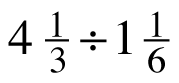2. 8.06 ÷ 2.48

3.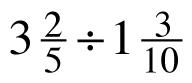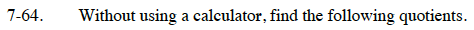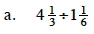For part (a), start by rewriting the mixed numbers as a fractions greater than one:

Now, instead of dividing the fractions, multiply the first fraction by the reciprocal of the second fraction.

Proceed by multiplying the numerators and the denominators and finish by simplifying the fraction.

$4\frac{1}{3}=\frac{13}{3}\ \text{and}\ 1\frac{1}{6}=\frac{7}{6}$

$\frac{13}{3}\div\frac{7}{6}=\frac{13}{3}\cdot\frac{6}{7}$

$\frac{13}{3}\cdot\frac{6}{7}=\frac{78}{21}$

$\frac{26}{7}$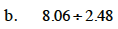For part (b), divide using long division.
Don't forget to move the decimal point in the quotient once you have divided!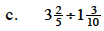For part (c), refer to the process outlined in part (a).